# Math Worksheets Land

Math Worksheets For All Ages

# Math Worksheets Land

Math Worksheets For All Ages

# Identify and Classify Angles Worksheets

Having the ability to communicate measures is one of key aspects of geometric relationships that we focus our attention to when working to understand geometric systems and structures of all kinds. One of core attributes of any shape is point where to lines meet called the vertex. The nature of that meeting results in all types of different angles being formed. We describe this meeting based on the measure of the angle that is created. In this section we will explore the naming and identification of these new various slants. We will key in on four main classifications. These worksheets and lessons will help students identify acute, right, straight, and obtuse angles. A complete explanation and even a little more is explained at the bottom of this page, if you could use a quick refresher on the subject matter.

### Aligned Standard: Grade 4 Geometry - 4.G.1

• Answer Keys - These are for all the unlocked materials above.

### Homework Sheets

A real mix of geometry in here for you. We review some related standards, too.

• Identify and Classify Angles Homework 1 - Is this angle acute, right, obtuse, or straight?
• Lines or Line Segments HW 2 - A line goes without end in both directions. A ray has 1 endpoint and goes without end in 1 direction. A line segment has 2 endpoints.
• Parallel or Perpendicular HW 3 - Parallel lines are lines on the same plane that can continue infinitely, but do not intersect (touch each other). If you check any point on parallel lines, they are always the same distance apart.

### Practice Worksheets

Once again, line segments and lines sneak in here. The other 2 sheets focus on angles though.

• Practice 1 - Classify Set Angles
• Practice 2 - What is this? a) a line, b) a line segment, or c) a ray
• Practice 3 - Classify the line set as intersecting, parallel or perpendicular.

### Math Skill Quizzes

All you will find here are angles and line sets.

• Quiz 1 - What is this? Do not let the end points throw you for a loop.
• Quiz 2 - Is this angle acute, right, obtuse, or straight?
• Quiz 3 - Label the line sets. Make use of any variable of your choosing. Just make sure to keep them consistent.

### What are the Classifications of Angles?

A major portion of mathematics is geometry. Geometry is the fundamental math that is used in the design of just about anything. It helps us determine the types of materials to use and how to arrange those materials when constructing most things. When two lines meet to form a point, an angle is created. Understanding how those lines are orientated can give us a major bit of insight into the strength of structures that they form. We often need to communicate the nature of these angles. There are five major terms that we use to classify angles to one another. They are all centered on the measure of the angle in degrees. If you face problems identifying the angles, here's a small list for based on the classification of angles:

Acute - This is an angle whose measures equals to more than 0° but less than 90°. These are your narrower angles. All angles with 30° , 40° , 60° are acute angles. You will see these in everyday things like slices of pizza and watermelon. Ramps and inclines share this property. An example is drawn below: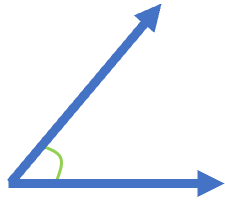Right - A right-angle has measure angles equal to 90°. One line points in a due North or South direction and the other line points directly East or West. You will see this on an analog clock at the 3 o'clock and 9 o'clock times. An example is drawn below: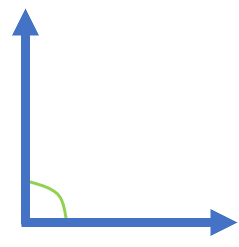Obtuse - This angle has a measure of more than 90° however, less than 180°. They are just past a right angle, but not quite straight. You will notice this is lounge chairs and boomerangs.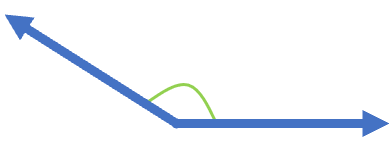Straight - When it comes to an angle whose measure equals 180° , it is called a straight angle. Which make sense because they are straight as well. The walls and floors of your classroom share this property. An example is drawn below: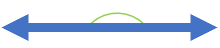Reflex - When it comes to an angle whose measure is greater than 180° ,but less than 360° it is called a reflex angle. We will explore this classification now, but it will have a much greater presence in future geometry units. An example is drawn below: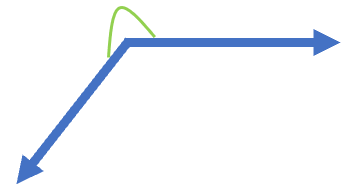Unlock all the answers, worksheets, homework, tests and more!
Save Tons of Time! Make My Life Easier Now

## Thanks and Don't Forget To Tell Your Friends!

I would appreciate everyone letting me know if you find any errors. I'm getting a little older these days and my eyes are going. Please contact me, to let me know. I'll fix it ASAP.